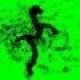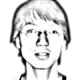# Generate Recursive Imagesratio = 0.45; pi = 759; pj = 1496; w = 1914; h = 1254; Nit = 100; threshold = 10;   img = imread('input.jpg'); x = img;   for iit = 1:Nit xsmall = imresize(x, ratio); xnew = img; xnew(pi:pi+h-1, pj:pj+w-1,:) = xsmall(1:h,1:w,:);   diff = x-xnew; diff = sum(sum(sum(diff.*diff))); if diff < threshold break; end   x = xnew; imwrite(x, sprintf('iter-%02d.jpg', iit), 'jpg'); end时，上式右边非负，且### 9 comments to Generate Recursive Images

•blackball

我在一年半以前想过这个问题，没有找到其理论依据，今天受益匪浅。谢谢

•maxint

sf没了，呜呜

•gaohaidong

以前我想过这个东西的。不过没像你而已。。。

•jon

你好！请问你上面的matlab程序我运行了会有错误的呢？是不是因为图片大小的原因？谢谢！

•pluskid

对呀，我偷懒直接在那里硬编码了图片的大小和一些位置坐标，请根据自己的需求做相应的修改。

•maxftd

请问下 diff = sum(sum(sum(diff.*diff)));这句是什么意思?
为什么他小于threshod就退出循环。。

•pluskid

就是两幅图像的像素之差的平方和啦

•maxftd

if diff < threshold
break;
end
这句的意图是神马？感觉没必要，

•pluskid

提前退出